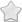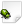OPEN  Crash report #1234  -  [A3892EA] debugHud_Audio() DebugHudAudio.cpp:40
Posted Jul 29, 2018 - updated Jul 29, 2018   Shortlink: http://arx.vg/1234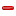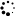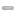0 Votes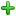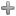Issue details
• Type of issue
Crash report
• Status
 New
• Assigned to
Not assigned to anyone
• Type of bug
Not triaged
• Likelihood
Not triaged
• Effect
Not triaged
• Posted by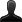CrashBot
• Owned by
Not owned by anyone
• Estimated time
Not estimated
• Category
Not determined
• Resolution
Not determined
• Priority
Not determined
• Reproducability
Not determined
• Severity
Not determined
• Targetted for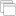Not determined
• OS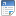Linux
• Architectureamd64
• Fixed inNot determined
Issue description
Illegal storage access: address not mapped to object

``` Instruction address: 0x562881f278d7
Memory accessed: 0x102c0000c
Stack pointer: 0x7ffc39e7bbe0
```

Callstack:
``` arx!0x562881f7b6ae
arx!0x562881f7b8d0
arx!0x562881f278d7
arx!0x562881cba0b8
arx!0x562881cba602
arx!0x562881cd6369
arx!0x562881c33c5f
libc-2.27.so!0x7f7338a719da
arx!0x562881c457ea
```

GDB stack trace:
``` [New LWP 11919]
[New LWP 11920]
[New LWP 11921]
[New LWP 11922]
[New LWP 11923]
[New LWP 11924]
[New LWP 11925]
[New LWP 11926]
[New LWP 11927]
[New LWP 11928]
[New LWP 11929]
[New LWP 11930]
[New LWP 11934]
[New LWP 11935]
[New LWP 11936]
[New LWP 12094]
0x00007f733a9baef8 in __GI___nanosleep (requested_time=requested_time@entry=0x7ffc39e7b5d0, remaining=remaining@entry=0x0) at ../sysdeps/unix/sysv/linux/nanosleep.c:28
Id   Target Id         Frame
* 1    Thread 0x7f733ad72b80 (LWP 11918) "arx" 0x00007f733a9baef8 in __GI___nanosleep (requested_time=requested_time@entry=0x7ffc39e7b5d0, remaining=remaining@entry=0x0) at ../sysdeps/unix/sysv/linux/nanosleep.c:28
2    Thread 0x7f732b497700 (LWP 11919) "arx:cs0" 0x00007f733a9b63fc in futex_wait_cancelable (private=<optimized out>, expected=0, futex_word=0x5628831910a8) at ../sysdeps/unix/sysv/linux/futex-internal.h:88
3    Thread 0x7f732a93f700 (LWP 11920) "arx:disk\$0" 0x00007f733a9b63fc in futex_wait_cancelable (private=<optimized out>, expected=0, futex_word=0x562883165be8) at ../sysdeps/unix/sysv/linux/futex-internal.h:88
4    Thread 0x7f732a13e700 (LWP 11921) "arx:sh0" 0x00007f733a9b63fc in futex_wait_cancelable (private=<optimized out>, expected=0, futex_word=0x562883193f6c) at ../sysdeps/unix/sysv/linux/futex-internal.h:88
5    Thread 0x7f732993d700 (LWP 11922) "arx:sh1" 0x00007f733a9b63fc in futex_wait_cancelable (private=<optimized out>, expected=0, futex_word=0x562883193f68) at ../sysdeps/unix/sysv/linux/futex-internal.h:88
6    Thread 0x7f732913c700 (LWP 11923) "arx:sh2" 0x00007f733a9b63fc in futex_wait_cancelable (private=<optimized out>, expected=0, futex_word=0x562883193f68) at ../sysdeps/unix/sysv/linux/futex-internal.h:88
7    Thread 0x7f732893b700 (LWP 11924) "arx:sh3" 0x00007f733a9b63fc in futex_wait_cancelable (private=<optimized out>, expected=0, futex_word=0x562883193f68) at ../sysdeps/unix/sysv/linux/futex-internal.h:88
8    Thread 0x7f7313fff700 (LWP 11925) "arx:sh4" 0x00007f733a9b63fc in futex_wait_cancelable (private=<optimized out>, expected=0, futex_word=0x562883193f6c) at ../sysdeps/unix/sysv/linux/futex-internal.h:88
9    Thread 0x7f73137fe700 (LWP 11926) "arx:sh5" 0x00007f733a9b63fc in futex_wait_cancelable (private=<optimized out>, expected=0, futex_word=0x562883193f68) at ../sysdeps/unix/sysv/linux/futex-internal.h:88
10   Thread 0x7f7312ffd700 (LWP 11927) "arx:shlo0" 0x00007f733a9b63fc in futex_wait_cancelable (private=<optimized out>, expected=0, futex_word=0x56288319436c) at ../sysdeps/unix/sysv/linux/futex-internal.h:88
11   Thread 0x7f73127fc700 (LWP 11928) "arx:shlo1" 0x00007f733a9b63fc in futex_wait_cancelable (private=<optimized out>, expected=0, futex_word=0x56288319436c) at ../sysdeps/unix/sysv/linux/futex-internal.h:88
12   Thread 0x7f7311ffb700 (LWP 11929) "arx:shlo2" 0x00007f733a9b63fc in futex_wait_cancelable (private=<optimized out>, expected=0, futex_word=0x56288319436c) at ../sysdeps/unix/sysv/linux/futex-internal.h:88
13   Thread 0x7f73117fa700 (LWP 11930) "arx:shlo3" 0x00007f733a9b63fc in futex_wait_cancelable (private=<optimized out>, expected=0, futex_word=0x562883194368) at ../sysdeps/unix/sysv/linux/futex-internal.h:88
14   Thread 0x7f7310ff9700 (LWP 11934) "arx:gdrv0" 0x00007f733a9b63fc in futex_wait_cancelable (private=<optimized out>, expected=0, futex_word=0x56288361aab4) at ../sysdeps/unix/sysv/linux/futex-internal.h:88
15   Thread 0x7f73107f8700 (LWP 11935) "arx" 0x00007f7338b45cb3 in __GI___poll (fds=fds@entry=0x7f73107f7dc0, nfds=nfds@entry=1, timeout=timeout@entry=1000) at ../sysdeps/unix/sysv/linux/poll.c:29
16   Thread 0x7f72f37a9700 (LWP 11936) "Sound Update" 0x00007f733a9baef8 in __GI___nanosleep (requested_time=requested_time@entry=0x7f72f37a8eb0, remaining=remaining@entry=0x0) at ../sysdeps/unix/sysv/linux/nanosleep.c:28
17   Thread 0x7f72f1a36700 (LWP 12094) "Pathfinder" 0x00007f733a9baef8 in __GI___nanosleep (requested_time=requested_time@entry=0x7f72f1a35e00, remaining=remaining@entry=0x0) at ../sysdeps/unix/sysv/linux/nanosleep.c:28

#0  0x00007f733a9baef8 in __GI___nanosleep (requested_time=requested_time@entry=0x7f72f1a35e00, remaining=remaining@entry=0x0) at ../sysdeps/unix/sysv/linux/nanosleep.c:28
resultvar = 18446744073709551100
sc_cancel_oldtype = 0
sc_ret = <optimized out>
t = {tv_sec = 0, tv_nsec = 10000000}
#2  0x0000562881c4b27f in PathFinderThread::run (this=0x562887423710) at /home/dscharrer/pro/arx/src/ai/PathFinderManager.cpp:139
eb = <optimized out>
pathfinder = {m_radius = 25, m_height = -45, m_heuristic = 0.21800001, map_s = 1867, map_d = 0x5628851e2a10, slight_c = 1200, slight_l = 0x5628825b7540 <g_staticLights>}
pd = 0x7f72f1a36700
now = <optimized out>
unwind_buf = {cancel_jmp_buf = {{jmp_buf = {140131657017088, -5828191192243239344, 140721279964654, 140721279964655, 140721279964656, 22, 5907589921560331856, 5906593819011057232}, mask_was_saved = 0}}, priv = {pad = {0x0, 0x0, 0x0, 0x0}, data = {prev = 0x0, cleanup = 0x0, canceltype = 0}}}
not_first_call = <optimized out>
#5  0x00007f7338b515ef in clone () at ../sysdeps/unix/sysv/linux/x86_64/clone.S:95
No locals.

#0  0x00007f733a9baef8 in __GI___nanosleep (requested_time=requested_time@entry=0x7f72f37a8eb0, remaining=remaining@entry=0x0) at ../sysdeps/unix/sysv/linux/nanosleep.c:28
resultvar = 18446744073709551100
sc_cancel_oldtype = 0
sc_ret = <optimized out>
t = {tv_sec = 0, tv_nsec = 100000000}
#2  0x0000562881fa404c in SoundUpdateThread::run (this=0x5628838f7640) at /home/dscharrer/pro/arx/src/scene/GameSound.cpp:1255
No locals.
pd = 0x7f72f37a9700
now = <optimized out>
unwind_buf = {cancel_jmp_buf = {{jmp_buf = {140131687896832, -5828191192243239344, 140721279976798, 140721279976799, 140721279976800, 0, 5907592448611714640, 5906593819011057232}, mask_was_saved = 0}}, priv = {pad = {0x0, 0x0, 0x0, 0x0}, data = {prev = 0x0, cleanup = 0x0, canceltype = 0}}}
not_first_call = <optimized out>
#5  0x00007f7338b515ef in clone () at ../sysdeps/unix/sysv/linux/x86_64/clone.S:95
No locals.

#0  0x00007f7338b45cb3 in __GI___poll (fds=fds@entry=0x7f73107f7dc0, nfds=nfds@entry=1, timeout=timeout@entry=1000) at ../sysdeps/unix/sysv/linux/poll.c:29
resultvar = 18446744073709551100
sc_cancel_oldtype = 0
sc_ret = <optimized out>
#1  0x00007f7338374eef in poll (__timeout=1000, __nfds=1, __fds=0x7f73107f7dc0) at /usr/include/bits/poll2.h:46
No locals.
#2  snd1_pcm_wait_nocheck (pcm=0x562883ac16c0, timeout=1000) at /var/tmp/portage/media-libs/alsa-lib-1.1.6-r1/work/alsa-lib-1.1.6/src/pcm/pcm.c:2792
pfd = 0x7f73107f7dc0
revents = 0
npfds = <optimized out>
err = <optimized out>
err_poll = <optimized out>
__FUNCTION__ = "snd1_pcm_wait_nocheck"
#3  0x00007f73383751ab in snd_pcm_wait (pcm=0x562883ac16c0, timeout=<optimized out>) at /var/tmp/portage/media-libs/alsa-lib-1.1.6-r1/work/alsa-lib-1.1.6/src/pcm/pcm.c:2743
err = <optimized out>
#4  0x00007f733a2900cc in ALCplaybackAlsa_mixerProc (ptr=ptr@entry=0x5628835a8640) at /var/tmp/portage/media-libs/openal-1.18.2-r1/work/openal-soft-1.18.2/Alc/backends/alsa.c:524
state = 3
self = 0x5628835a8640
device = 0x5628838ff380
areas = 0x562883ac0e10
update_size = 1024
avail = <optimized out>
commitres = <optimized out>
offset = 2048
frames = 1024
WritePtr = <optimized out>
err = <optimized out>
__FUNCTION__ = "ALCplaybackAlsa_mixerProc"
#5  0x00007f733a252ae7 in althrd_starter (arg=<optimized out>) at /var/tmp/portage/media-libs/openal-1.18.2-r1/work/openal-soft-1.18.2/common/threads.c:528
cntr = <optimized out>
pd = 0x7f73107f8700
now = <optimized out>
unwind_buf = {cancel_jmp_buf = {{jmp_buf = {140132174759680, -5828191192243239344, 140721279974990, 140721279974991, 140721279975136, 0, 5906534698561570384, 5906593819011057232}, mask_was_saved = 0}}, priv = {pad = {0x0, 0x0, 0x0, 0x0}, data = {prev = 0x0, cleanup = 0x0, canceltype = 0}}}
not_first_call = <optimized out>
#7  0x00007f7338b515ef in clone () at ../sysdeps/unix/sysv/linux/x86_64/clone.S:95
No locals.

#0  0x00007f733a9b63fc in futex_wait_cancelable (private=<optimized out>, expected=0, futex_word=0x56288361aab4) at ../sysdeps/unix/sysv/linux/futex-internal.h:88
__ret = -512
oldtype = 0
err = <optimized out>
oldtype = <optimized out>
err = <optimized out>
__ret = <optimized out>
resultvar = <optimized out>
__arg4 = <optimized out>
__arg3 = <optimized out>
__arg2 = <optimized out>
__arg1 = <optimized out>
_a4 = <optimized out>
_a3 = <optimized out>
_a2 = <optimized out>
_a1 = <optimized out>
spin = 0
buffer = {__routine = 0x7f733a9b6150 <__condvar_cleanup_waiting>, __arg = 0x7f7310ff8e00, __canceltype = -2095816112, __prev = 0x0}
cbuffer = {wseq = 1147019, cond = 0x56288361aa88, mutex = 0x56288361aa60, private = 0}
rt = <optimized out>
err = <optimized out>
g = 1
flags = <optimized out>
g1_start = <optimized out>
signals = <optimized out>
result = 0
wseq = <optimized out>
seq = 573509
private = <optimized out>
maxspin = <optimized out>
err = <optimized out>
result = <optimized out>
wseq = <optimized out>
g = <optimized out>
seq = <optimized out>
flags = <optimized out>
private = <optimized out>
signals = <optimized out>
g1_start = <optimized out>
spin = <optimized out>
buffer = <optimized out>
cbuffer = <optimized out>
rt = <optimized out>
s = <optimized out>
No locals.
#3  0x00007f733431f7ea in cnd_wait (mtx=0x56288361aa60, cond=0x56288361aa88) at ../mesa-9999/src/../include/c11/threads_posix.h:155
No locals.
job = <optimized out>
queue = 0x56288361aa28
#5  0x00007f733431f51f in impl_thrd_routine (p=<optimized out>) at ../mesa-9999/src/../include/c11/threads_posix.h:87
pack = {func = 0x7f733431f750 <util_queue_thread_func>, arg = 0x562883637c70}
pd = 0x7f7310ff9700
now = <optimized out>
unwind_buf = {cancel_jmp_buf = {{jmp_buf = {140132183152384, -5828191192243239344, 140721279972462, 140721279972463, 140721279972464, 22, 5906535792167618128, 5906593819011057232}, mask_was_saved = 0}}, priv = {pad = {0x0, 0x0, 0x0, 0x0}, data = {prev = 0x0, cleanup = 0x0, canceltype = 0}}}
not_first_call = <optimized out>
#7  0x00007f7338b515ef in clone () at ../sysdeps/unix/sysv/linux/x86_64/clone.S:95
No locals.

#0  0x00007f733a9b63fc in futex_wait_cancelable (private=<optimized out>, expected=0, futex_word=0x562883194368) at ../sysdeps/unix/sysv/linux/futex-internal.h:88
__ret = -512
oldtype = 0
err = <optimized out>
oldtype = <optimized out>
err = <optimized out>
__ret = <optimized out>
resultvar = <optimized out>
__arg4 = <optimized out>
__arg3 = <optimized out>
__arg2 = <optimized out>
__arg1 = <optimized out>
_a4 = <optimized out>
_a3 = <optimized out>
_a2 = <optimized out>
_a1 = <optimized out>
spin = 0
buffer = {__routine = 0x7f733a9b6150 <__condvar_cleanup_waiting>, __arg = 0x7f73117f9e00, __canceltype = 11264, __prev = 0x0}
cbuffer = {wseq = 16, cond = 0x562883194340, mutex = 0x562883194318, private = 0}
rt = <optimized out>
err = <optimized out>
g = 0
flags = <optimized out>
g1_start = <optimized out>
signals = <optimized out>
result = 0
wseq = <optimized out>
seq = 8
private = <optimized out>
maxspin = <optimized out>
err = <optimized out>
result = <optimized out>
wseq = <optimized out>
g = <optimized out>
seq = <optimized out>
flags = <optimized out>
private = <optimized out>
signals = <optimized out>
g1_start = <optimized out>
spin = <optimized out>
buffer = <optimized out>
cbuffer = <optimized out>
rt = <optimized out>
s = <optimized out>
No locals.
#3  0x00007f733431f7ea in cnd_wait (mtx=0x562883194318, cond=0x562883194340) at ../mesa-9999/src/../include/c11/threads_posix.h:155
No locals.
job = <optimized out>
queue = 0x5628831942e0
#5  0x00007f733431f51f in impl_thrd_routine (p=<optimized out>) at ../mesa-9999/src/../include/c11/threads_posix.h:87
pack = {func = 0x7f733431f750 <util_queue_thread_func>, arg = 0x562883197460}
pd = 0x7f73117fa700
now = <optimized out>
unwind_buf = {cancel_jmp_buf = {{jmp_buf = {140132191545088, -5828191192243239344, 140721279971630, 140721279971631, 140721279971632, 0, 5906532494169605712, 5906593819011057232}, mask_was_saved = 0}}, priv = {pad = {0x0, 0x0, 0x0, 0x0}, data = {prev = 0x0, cleanup = 0x0, canceltype = 0}}}
not_first_call = <optimized out>
#7  0x00007f7338b515ef in clone () at ../sysdeps/unix/sysv/linux/x86_64/clone.S:95
No locals.

#0  0x00007f733a9b63fc in futex_wait_cancelable (private=<optimized out>, expected=0, futex_word=0x56288319436c) at ../sysdeps/unix/sysv/linux/futex-internal.h:88
__ret = -512
oldtype = 0
err = <optimized out>
oldtype = <optimized out>
err = <optimized out>
__ret = <optimized out>
resultvar = <optimized out>
__arg4 = <optimized out>
__arg3 = <optimized out>
__arg2 = <optimized out>
__arg1 = <optimized out>
_a4 = <optimized out>
_a3 = <optimized out>
_a2 = <optimized out>
_a1 = <optimized out>
spin = 0
buffer = {__routine = 0x7f733a9b6150 <__condvar_cleanup_waiting>, __arg = 0x7f7311ffae00, __canceltype = 624, __prev = 0x0}
cbuffer = {wseq = 13, cond = 0x562883194340, mutex = 0x562883194318, private = 0}
rt = <optimized out>
err = <optimized out>
g = 1
flags = <optimized out>
g1_start = <optimized out>
signals = <optimized out>
result = 0
wseq = <optimized out>
seq = 6
private = <optimized out>
maxspin = <optimized out>
err = <optimized out>
result = <optimized out>
wseq = <optimized out>
g = <optimized out>
seq = <optimized out>
flags = <optimized out>
private = <optimized out>
signals = <optimized out>
g1_start = <optimized out>
spin = <optimized out>
buffer = <optimized out>
cbuffer = <optimized out>
rt = <optimized out>
s = <optimized out>
No locals.
#3  0x00007f733431f7ea in cnd_wait (mtx=0x562883194318, cond=0x562883194340) at ../mesa-9999/src/../include/c11/threads_posix.h:155
No locals.
job = <optimized out>
queue = 0x5628831942e0
#5  0x00007f733431f51f in impl_thrd_routine (p=<optimized out>) at ../mesa-9999/src/../include/c11/threads_posix.h:87
pack = {func = 0x7f733431f750 <util_queue_thread_func>, arg = 0x562883197290}
pd = 0x7f7311ffb700
now = <optimized out>
unwind_buf = {cancel_jmp_buf = {{jmp_buf = {140132199937792, -5828191192243239344, 140721279971630, 140721279971631, 140721279971632, 0, 5906533592070620752, 5906593819011057232}, mask_was_saved = 0}}, priv = {pad = {0x0, 0x0, 0x0, 0x0}, data = {prev = 0x0, cleanup = 0x0, canceltype = 0}}}
not_first_call = <optimized out>
#7  0x00007f7338b515ef in clone () at ../sysdeps/unix/sysv/linux/x86_64/clone.S:95
No locals.

#0  0x00007f733a9b63fc in futex_wait_cancelable (private=<optimized out>, expected=0, futex_word=0x56288319436c) at ../sysdeps/unix/sysv/linux/futex-internal.h:88
__ret = -512
oldtype = 0
err = <optimized out>
oldtype = <optimized out>
err = <optimized out>
__ret = <optimized out>
resultvar = <optimized out>
__arg4 = <optimized out>
__arg3 = <optimized out>
__arg2 = <optimized out>
__arg1 = <optimized out>
_a4 = <optimized out>
_a3 = <optimized out>
_a2 = <optimized out>
_a1 = <optimized out>
spin = 0
buffer = {__routine = 0x7f733a9b6150 <__condvar_cleanup_waiting>, __arg = 0x7f73127fbe00, __canceltype = 624, __prev = 0x0}
cbuffer = {wseq = 15, cond = 0x562883194340, mutex = 0x562883194318, private = 0}
rt = <optimized out>
err = <optimized out>
g = 1
flags = <optimized out>
g1_start = <optimized out>
signals = <optimized out>
result = 0
wseq = <optimized out>
seq = 7
private = <optimized out>
maxspin = <optimized out>
err = <optimized out>
result = <optimized out>
wseq = <optimized out>
g = <optimized out>
seq = <optimized out>
flags = <optimized out>
private = <optimized out>
signals = <optimized out>
g1_start = <optimized out>
spin = <optimized out>
buffer = <optimized out>
cbuffer = <optimized out>
rt = <optimized out>
s = <optimized out>
No locals.
#3  0x00007f733431f7ea in cnd_wait (mtx=0x562883194318, cond=0x562883194340) at ../mesa-9999/src/../include/c11/threads_posix.h:155
No locals.
job = <optimized out>
queue = 0x5628831942e0
#5  0x00007f733431f51f in impl_thrd_routine (p=<optimized out>) at ../mesa-9999/src/../include/c11/threads_posix.h:87
pack = {func = 0x7f733431f750 <util_queue_thread_func>, arg = 0x5628831970c0}
pd = 0x7f73127fc700
now = <optimized out>
unwind_buf = {cancel_jmp_buf = {{jmp_buf = {140132208330496, -5828191192243239344, 140721279971630, 140721279971631, 140721279971632, 0, 5906539090165630544, 5906593819011057232}, mask_was_saved = 0}}, priv = {pad = {0x0, 0x0, 0x0, 0x0}, data = {prev = 0x0, cleanup = 0x0, canceltype = 0}}}
not_first_call = <optimized out>
#7  0x00007f7338b515ef in clone () at ../sysdeps/unix/sysv/linux/x86_64/clone.S:95
No locals.

#0  0x00007f733a9b63fc in futex_wait_cancelable (private=<optimized out>, expected=0, futex_word=0x56288319436c) at ../sysdeps/unix/sysv/linux/futex-internal.h:88
__ret = -512
oldtype = 0
err = <optimized out>
oldtype = <optimized out>
err = <optimized out>
__ret = <optimized out>
resultvar = <optimized out>
__arg4 = <optimized out>
__arg3 = <optimized out>
__arg2 = <optimized out>
__arg1 = <optimized out>
_a4 = <optimized out>
_a3 = <optimized out>
_a2 = <optimized out>
_a1 = <optimized out>
spin = 0
buffer = {__routine = 0x7f733a9b6150 <__condvar_cleanup_waiting>, __arg = 0x7f7312ffce00, __canceltype = 624, __prev = 0x0}
cbuffer = {wseq = 11, cond = 0x562883194340, mutex = 0x562883194318, private = 0}
rt = <optimized out>
err = <optimized out>
g = 1
flags = <optimized out>
g1_start = <optimized out>
signals = <optimized out>
result = 0
wseq = <optimized out>
seq = 5
private = <optimized out>
maxspin = <optimized out>
err = <optimized out>
result = <optimized out>
wseq = <optimized out>
g = <optimized out>
seq = <optimized out>
flags = <optimized out>
private = <optimized out>
signals = <optimized out>
g1_start = <optimized out>
spin = <optimized out>
buffer = <optimized out>
cbuffer = <optimized out>
rt = <optimized out>
s = <optimized out>
No locals.
#3  0x00007f733431f7ea in cnd_wait (mtx=0x562883194318, cond=0x562883194340) at ../mesa-9999/src/../include/c11/threads_posix.h:155
No locals.
job = <optimized out>
queue = 0x5628831942e0
#5  0x00007f733431f51f in impl_thrd_routine (p=<optimized out>) at ../mesa-9999/src/../include/c11/threads_posix.h:87
pack = {func = 0x7f733431f750 <util_queue_thread_func>, arg = 0x56288318fa20}
pd = 0x7f7312ffd700
now = <optimized out>
unwind_buf = {cancel_jmp_buf = {{jmp_buf = {140132216723200, -5828191192243239344, 140721279971630, 140721279971631, 140721279971632, 0, 5906540192361612880, 5906593819011057232}, mask_was_saved = 0}}, priv = {pad = {0x0, 0x0, 0x0, 0x0}, data = {prev = 0x0, cleanup = 0x0, canceltype = 0}}}
not_first_call = <optimized out>
#7  0x00007f7338b515ef in clone () at ../sysdeps/unix/sysv/linux/x86_64/clone.S:95
No locals.

#0  0x00007f733a9b63fc in futex_wait_cancelable (private=<optimized out>, expected=0, futex_word=0x562883193f68) at ../sysdeps/unix/sysv/linux/futex-internal.h:88
__ret = -512
oldtype = 0
err = <optimized out>
oldtype = <optimized out>
err = <optimized out>
__ret = <optimized out>
resultvar = <optimized out>
__arg4 = <optimized out>
__arg3 = <optimized out>
__arg2 = <optimized out>
__arg1 = <optimized out>
_a4 = <optimized out>
_a3 = <optimized out>
_a2 = <optimized out>
_a1 = <optimized out>
spin = 0
buffer = {__routine = 0x7f733a9b6150 <__condvar_cleanup_waiting>, __arg = 0x7f73137fde00, __canceltype = 0, __prev = 0x0}
cbuffer = {wseq = 70, cond = 0x562883193f40, mutex = 0x562883193f18, private = 0}
rt = <optimized out>
err = <optimized out>
g = 0
flags = <optimized out>
g1_start = <optimized out>
signals = <optimized out>
result = 0
wseq = <optimized out>
seq = 35
private = <optimized out>
maxspin = <optimized out>
err = <optimized out>
result = <optimized out>
wseq = <optimized out>
g = <optimized out>
seq = <optimized out>
flags = <optimized out>
private = <optimized out>
signals = <optimized out>
g1_start = <optimized out>
spin = <optimized out>
buffer = <optimized out>
cbuffer = <optimized out>
rt = <optimized out>
s = <optimized out>
No locals.
#3  0x00007f733431f7ea in cnd_wait (mtx=0x562883193f18, cond=0x562883193f40) at ../mesa-9999/src/../include/c11/threads_posix.h:155
No locals.
job = <optimized out>
queue = 0x562883193ee0
#5  0x00007f733431f51f in impl_thrd_routine (p=<optimized out>) at ../mesa-9999/src/../include/c11/threads_posix.h:87
pack = {func = 0x7f733431f750 <util_queue_thread_func>, arg = 0x562883196550}
pd = 0x7f73137fe700
now = <optimized out>
unwind_buf = {cancel_jmp_buf = {{jmp_buf = {140132225115904, -5828191192243239344, 140721279971630, 140721279971631, 140721279971632, 0, 5906536894363600464, 5906593819011057232}, mask_was_saved = 0}}, priv = {pad = {0x0, 0x0, 0x0, 0x0}, data = {prev = 0x0, cleanup = 0x0, canceltype = 0}}}
not_first_call = <optimized out>
#7  0x00007f7338b515ef in clone () at ../sysdeps/unix/sysv/linux/x86_64/clone.S:95
No locals.

#0  0x00007f733a9b63fc in futex_wait_cancelable (private=<optimized out>, expected=0, futex_word=0x562883193f6c) at ../sysdeps/unix/sysv/linux/futex-internal.h:88
__ret = -512
oldtype = 0
err = <optimized out>
oldtype = <optimized out>
err = <optimized out>
__ret = <optimized out>
resultvar = <optimized out>
__arg4 = <optimized out>
__arg3 = <optimized out>
__arg2 = <optimized out>
__arg1 = <optimized out>
_a4 = <optimized out>
_a3 = <optimized out>
_a2 = <optimized out>
_a1 = <optimized out>
spin = 0
buffer = {__routine = 0x7f733a9b6150 <__condvar_cleanup_waiting>, __arg = 0x7f7313ffee00, __canceltype = 0, __prev = 0x0}
cbuffer = {wseq = 75, cond = 0x562883193f40, mutex = 0x562883193f18, private = 0}
rt = <optimized out>
err = <optimized out>
g = 1
flags = <optimized out>
g1_start = <optimized out>
signals = <optimized out>
result = 0
wseq = <optimized out>
seq = 37
private = <optimized out>
maxspin = <optimized out>
err = <optimized out>
result = <optimized out>
wseq = <optimized out>
g = <optimized out>
seq = <optimized out>
flags = <optimized out>
private = <optimized out>
signals = <optimized out>
g1_start = <optimized out>
spin = <optimized out>
buffer = <optimized out>
cbuffer = <optimized out>
rt = <optimized out>
s = <optimized out>
No locals.
#3  0x00007f733431f7ea in cnd_wait (mtx=0x562883193f18, cond=0x562883193f40) at ../mesa-9999/src/../include/c11/threads_posix.h:155
No locals.
job = <optimized out>
queue = 0x562883193ee0
#5  0x00007f733431f51f in impl_thrd_routine (p=<optimized out>) at ../mesa-9999/src/../include/c11/threads_posix.h:87
pack = {func = 0x7f733431f750 <util_queue_thread_func>, arg = 0x562883196380}
pd = 0x7f7313fff700
now = <optimized out>
unwind_buf = {cancel_jmp_buf = {{jmp_buf = {140132233508608, -5828191192243239344, 140721279971630, 140721279971631, 140721279971632, 0, 5906537992264615504, 5906593819011057232}, mask_was_saved = 0}}, priv = {pad = {0x0, 0x0, 0x0, 0x0}, data = {prev = 0x0, cleanup = 0x0, canceltype = 0}}}
not_first_call = <optimized out>
#7  0x00007f7338b515ef in clone () at ../sysdeps/unix/sysv/linux/x86_64/clone.S:95
No locals.

#0  0x00007f733a9b63fc in futex_wait_cancelable (private=<optimized out>, expected=0, futex_word=0x562883193f68) at ../sysdeps/unix/sysv/linux/futex-internal.h:88
__ret = -512
oldtype = 0
err = <optimized out>
oldtype = <optimized out>
err = <optimized out>
__ret = <optimized out>
resultvar = <optimized out>
__arg4 = <optimized out>
__arg3 = <optimized out>
__arg2 = <optimized out>
__arg1 = <optimized out>
_a4 = <optimized out>
_a3 = <optimized out>
_a2 = <optimized out>
_a1 = <optimized out>
spin = 0
buffer = {__routine = 0x7f733a9b6150 <__condvar_cleanup_waiting>, __arg = 0x7f732893ae00, __canceltype = 0, __prev = 0x0}
cbuffer = {wseq = 68, cond = 0x562883193f40, mutex = 0x562883193f18, private = 0}
rt = <optimized out>
err = <optimized out>
g = 0
flags = <optimized out>
g1_start = <optimized out>
signals = <optimized out>
result = 0
wseq = <optimized out>
seq = 34
private = <optimized out>
maxspin = <optimized out>
err = <optimized out>
result = <optimized out>
wseq = <optimized out>
g = <optimized out>
seq = <optimized out>
flags = <optimized out>
private = <optimized out>
signals = <optimized out>
g1_start = <optimized out>
spin = <optimized out>
buffer = <optimized out>
cbuffer = <optimized out>
rt = <optimized out>
s = <optimized out>
No locals.
#3  0x00007f733431f7ea in cnd_wait (mtx=0x562883193f18, cond=0x562883193f40) at ../mesa-9999/src/../include/c11/threads_posix.h:155
No locals.
job = <optimized out>
queue = 0x562883193ee0
#5  0x00007f733431f51f in impl_thrd_routine (p=<optimized out>) at ../mesa-9999/src/../include/c11/threads_posix.h:87
pack = {func = 0x7f733431f750 <util_queue_thread_func>, arg = 0x5628831961b0}
pd = 0x7f732893b700
now = <optimized out>
unwind_buf = {cancel_jmp_buf = {{jmp_buf = {140132578735872, -5828191192243239344, 140721279971630, 140721279971631, 140721279971632, 0, 5906554310992856656, 5906593819011057232}, mask_was_saved = 0}}, priv = {pad = {0x0, 0x0, 0x0, 0x0}, data = {prev = 0x0, cleanup = 0x0, canceltype = 0}}}
not_first_call = <optimized out>
#7  0x00007f7338b515ef in clone () at ../sysdeps/unix/sysv/linux/x86_64/clone.S:95
No locals.

#0  0x00007f733a9b63fc in futex_wait_cancelable (private=<optimized out>, expected=0, futex_word=0x562883193f68) at ../sysdeps/unix/sysv/linux/futex-internal.h:88
__ret = -512
oldtype = 0
err = <optimized out>
oldtype = <optimized out>
err = <optimized out>
__ret = <optimized out>
resultvar = <optimized out>
__arg4 = <optimized out>
__arg3 = <optimized out>
__arg2 = <optimized out>
__arg1 = <optimized out>
_a4 = <optimized out>
_a3 = <optimized out>
_a2 = <optimized out>
_a1 = <optimized out>
spin = 0
buffer = {__routine = 0x7f733a9b6150 <__condvar_cleanup_waiting>, __arg = 0x7f732913be00, __canceltype = 0, __prev = 0x0}
cbuffer = {wseq = 66, cond = 0x562883193f40, mutex = 0x562883193f18, private = 0}
rt = <optimized out>
err = <optimized out>
g = 0
flags = <optimized out>
g1_start = <optimized out>
signals = <optimized out>
result = 0
wseq = <optimized out>
seq = 33
private = <optimized out>
maxspin = <optimized out>
err = <optimized out>
result = <optimized out>
wseq = <optimized out>
g = <optimized out>
seq = <optimized out>
flags = <optimized out>
private = <optimized out>
signals = <optimized out>
g1_start = <optimized out>
spin = <optimized out>
buffer = <optimized out>
cbuffer = <optimized out>
rt = <optimized out>
s = <optimized out>
No locals.
#3  0x00007f733431f7ea in cnd_wait (mtx=0x562883193f18, cond=0x562883193f40) at ../mesa-9999/src/../include/c11/threads_posix.h:155
No locals.
job = <optimized out>
queue = 0x562883193ee0
#5  0x00007f733431f51f in impl_thrd_routine (p=<optimized out>) at ../mesa-9999/src/../include/c11/threads_posix.h:87
pack = {func = 0x7f733431f750 <util_queue_thread_func>, arg = 0x56288318f9b0}
pd = 0x7f732913c700
now = <optimized out>
unwind_buf = {cancel_jmp_buf = {{jmp_buf = {140132587128576, -5828191192243239344, 140721279971630, 140721279971631, 140721279971632, 0, 5906551012994844240, 5906593819011057232}, mask_was_saved = 0}}, priv = {pad = {0x0, 0x0, 0x0, 0x0}, data = {prev = 0x0, cleanup = 0x0, canceltype = 0}}}
not_first_call = <optimized out>
#7  0x00007f7338b515ef in clone () at ../sysdeps/unix/sysv/linux/x86_64/clone.S:95
No locals.

#0  0x00007f733a9b63fc in futex_wait_cancelable (private=<optimized out>, expected=0, futex_word=0x562883193f68) at ../sysdeps/unix/sysv/linux/futex-internal.h:88
__ret = -512
oldtype = 0
err = <optimized out>
oldtype = <optimized out>
err = <optimized out>
__ret = <optimized out>
resultvar = <optimized out>
__arg4 = <optimized out>
__arg3 = <optimized out>
__arg2 = <optimized out>
__arg1 = <optimized out>
_a4 = <optimized out>
_a3 = <optimized out>
_a2 = <optimized out>
_a1 = <optimized out>
spin = 0
buffer = {__routine = 0x7f733a9b6150 <__condvar_cleanup_waiting>, __arg = 0x7f732993ce00, __canceltype = 0, __prev = 0x0}
cbuffer = {wseq = 64, cond = 0x562883193f40, mutex = 0x562883193f18, private = 0}
rt = <optimized out>
err = <optimized out>
g = 0
flags = <optimized out>
g1_start = <optimized out>
signals = <optimized out>
result = 0
wseq = <optimized out>
seq = 32
private = <optimized out>
maxspin = <optimized out>
err = <optimized out>
result = <optimized out>
wseq = <optimized out>
g = <optimized out>
seq = <optimized out>
flags = <optimized out>
private = <optimized out>
signals = <optimized out>
g1_start = <optimized out>
spin = <optimized out>
buffer = <optimized out>
cbuffer = <optimized out>
rt = <optimized out>
s = <optimized out>
No locals.
#3  0x00007f733431f7ea in cnd_wait (mtx=0x562883193f18, cond=0x562883193f40) at ../mesa-9999/src/../include/c11/threads_posix.h:155
No locals.
job = <optimized out>
queue = 0x562883193ee0
#5  0x00007f733431f51f in impl_thrd_routine (p=<optimized out>) at ../mesa-9999/src/../include/c11/threads_posix.h:87
pack = {func = 0x7f733431f750 <util_queue_thread_func>, arg = 0x56288318f7e0}
pd = 0x7f732993d700
now = <optimized out>
unwind_buf = {cancel_jmp_buf = {{jmp_buf = {140132595521280, -5828191192243239344, 140721279971630, 140721279971631, 140721279971632, 0, 5906552115190826576, 5906593819011057232}, mask_was_saved = 0}}, priv = {pad = {0x0, 0x0, 0x0, 0x0}, data = {prev = 0x0, cleanup = 0x0, canceltype = 0}}}
not_first_call = <optimized out>
#7  0x00007f7338b515ef in clone () at ../sysdeps/unix/sysv/linux/x86_64/clone.S:95
No locals.

#0  0x00007f733a9b63fc in futex_wait_cancelable (private=<optimized out>, expected=0, futex_word=0x562883193f6c) at ../sysdeps/unix/sysv/linux/futex-internal.h:88
__ret = -512
oldtype = 0
err = <optimized out>
oldtype = <optimized out>
err = <optimized out>
__ret = <optimized out>
resultvar = <optimized out>
__arg4 = <optimized out>
__arg3 = <optimized out>
__arg2 = <optimized out>
__arg1 = <optimized out>
_a4 = <optimized out>
_a3 = <optimized out>
_a2 = <optimized out>
_a1 = <optimized out>
spin = 0
buffer = {__routine = 0x7f733a9b6150 <__condvar_cleanup_waiting>, __arg = 0x7f732a13de00, __canceltype = 0, __prev = 0x0}
cbuffer = {wseq = 73, cond = 0x562883193f40, mutex = 0x562883193f18, private = 0}
rt = <optimized out>
err = <optimized out>
g = 1
flags = <optimized out>
g1_start = <optimized out>
signals = <optimized out>
result = 0
wseq = <optimized out>
seq = 36
private = <optimized out>
maxspin = <optimized out>
err = <optimized out>
result = <optimized out>
wseq = <optimized out>
g = <optimized out>
seq = <optimized out>
flags = <optimized out>
private = <optimized out>
signals = <optimized out>
g1_start = <optimized out>
spin = <optimized out>
buffer = <optimized out>
cbuffer = <optimized out>
rt = <optimized out>
s = <optimized out>
No locals.
#3  0x00007f733431f7ea in cnd_wait (mtx=0x562883193f18, cond=0x562883193f40) at ../mesa-9999/src/../include/c11/threads_posix.h:155
No locals.
job = <optimized out>
queue = 0x562883193ee0
#5  0x00007f733431f51f in impl_thrd_routine (p=<optimized out>) at ../mesa-9999/src/../include/c11/threads_posix.h:87
pack = {func = 0x7f733431f750 <util_queue_thread_func>, arg = 0x56288318f610}
pd = 0x7f732a13e700
now = <optimized out>
unwind_buf = {cancel_jmp_buf = {{jmp_buf = {140132603913984, -5828191192243239344, 140721279971630, 140721279971631, 140721279971632, 0, 5906557613285836368, 5906593819011057232}, mask_was_saved = 0}}, priv = {pad = {0x0, 0x0, 0x0, 0x0}, data = {prev = 0x0, cleanup = 0x0, canceltype = 0}}}
not_first_call = <optimized out>
#7  0x00007f7338b515ef in clone () at ../sysdeps/unix/sysv/linux/x86_64/clone.S:95
No locals.

#0  0x00007f733a9b63fc in futex_wait_cancelable (private=<optimized out>, expected=0, futex_word=0x562883165be8) at ../sysdeps/unix/sysv/linux/futex-internal.h:88
__ret = -512
oldtype = 0
err = <optimized out>
oldtype = <optimized out>
err = <optimized out>
__ret = <optimized out>
resultvar = <optimized out>
__arg4 = <optimized out>
__arg3 = <optimized out>
__arg2 = <optimized out>
__arg1 = <optimized out>
_a4 = <optimized out>
_a3 = <optimized out>
_a2 = <optimized out>
_a1 = <optimized out>
spin = 0
buffer = {__routine = 0x7f733a9b6150 <__condvar_cleanup_waiting>, __arg = 0x7f732a93ee00, __canceltype = 624, __prev = 0x0}
cbuffer = {wseq = 0, cond = 0x562883165bc0, mutex = 0x562883165b98, private = 0}
rt = <optimized out>
err = <optimized out>
g = 0
flags = <optimized out>
g1_start = <optimized out>
signals = <optimized out>
result = 0
wseq = <optimized out>
seq = 0
private = <optimized out>
maxspin = <optimized out>
err = <optimized out>
result = <optimized out>
wseq = <optimized out>
g = <optimized out>
seq = <optimized out>
flags = <optimized out>
private = <optimized out>
signals = <optimized out>
g1_start = <optimized out>
spin = <optimized out>
buffer = <optimized out>
cbuffer = <optimized out>
rt = <optimized out>
s = <optimized out>
No locals.
#3  0x00007f733431f7ea in cnd_wait (mtx=0x562883165b98, cond=0x562883165bc0) at ../mesa-9999/src/../include/c11/threads_posix.h:155
No locals.
job = <optimized out>
queue = 0x562883165b60
#5  0x00007f733431f51f in impl_thrd_routine (p=<optimized out>) at ../mesa-9999/src/../include/c11/threads_posix.h:87
pack = {func = 0x7f733431f750 <util_queue_thread_func>, arg = 0x5628831952c0}
pd = 0x7f732a93f700
now = <optimized out>
unwind_buf = {cancel_jmp_buf = {{jmp_buf = {140132612306688, -5828191192243239344, 140721279971070, 140721279971071, 140721279971072, 0, 5906558711186851408, 5906593819011057232}, mask_was_saved = 0}}, priv = {pad = {0x0, 0x0, 0x0, 0x0}, data = {prev = 0x0, cleanup = 0x0, canceltype = 0}}}
not_first_call = <optimized out>
#7  0x00007f7338b515ef in clone () at ../sysdeps/unix/sysv/linux/x86_64/clone.S:95
No locals.

#0  0x00007f733a9b63fc in futex_wait_cancelable (private=<optimized out>, expected=0, futex_word=0x5628831910a8) at ../sysdeps/unix/sysv/linux/futex-internal.h:88
__ret = -512
oldtype = 0
err = <optimized out>
oldtype = <optimized out>
err = <optimized out>
__ret = <optimized out>
resultvar = <optimized out>
__arg4 = <optimized out>
__arg3 = <optimized out>
__arg2 = <optimized out>
__arg1 = <optimized out>
_a4 = <optimized out>
_a3 = <optimized out>
_a2 = <optimized out>
_a1 = <optimized out>
spin = 0
buffer = {__routine = 0x7f733a9b6150 <__condvar_cleanup_waiting>, __arg = 0x7f732b496e00, __canceltype = 3, __prev = 0x0}
cbuffer = {wseq = 363040, cond = 0x562883191080, mutex = 0x562883191058, private = 0}
rt = <optimized out>
err = <optimized out>
g = 0
flags = <optimized out>
g1_start = <optimized out>
signals = <optimized out>
result = 0
wseq = <optimized out>
seq = 181520
private = <optimized out>
maxspin = <optimized out>
err = <optimized out>
result = <optimized out>
wseq = <optimized out>
g = <optimized out>
seq = <optimized out>
flags = <optimized out>
private = <optimized out>
signals = <optimized out>
g1_start = <optimized out>
spin = <optimized out>
buffer = <optimized out>
cbuffer = <optimized out>
rt = <optimized out>
s = <optimized out>
No locals.
#3  0x00007f733431f7ea in cnd_wait (mtx=0x562883191058, cond=0x562883191080) at ../mesa-9999/src/../include/c11/threads_posix.h:155
No locals.
job = <optimized out>
queue = 0x562883191020
#5  0x00007f733431f51f in impl_thrd_routine (p=<optimized out>) at ../mesa-9999/src/../include/c11/threads_posix.h:87
pack = {func = 0x7f733431f750 <util_queue_thread_func>, arg = 0x56288318e620}
pd = 0x7f732b497700
now = <optimized out>
unwind_buf = {cancel_jmp_buf = {{jmp_buf = {140132624201472, -5828191192243239344, 140721279971790, 140721279971791, 140721279971792, 0, 5906554953090467408, 5906593819011057232}, mask_was_saved = 0}}, priv = {pad = {0x0, 0x0, 0x0, 0x0}, data = {prev = 0x0, cleanup = 0x0, canceltype = 0}}}
not_first_call = <optimized out>
#7  0x00007f7338b515ef in clone () at ../sysdeps/unix/sysv/linux/x86_64/clone.S:95
No locals.

#0  0x00007f733a9baef8 in __GI___nanosleep (requested_time=requested_time@entry=0x7ffc39e7b5d0, remaining=remaining@entry=0x0) at ../sysdeps/unix/sysv/linux/nanosleep.c:28
resultvar = 18446744073709551100
sc_cancel_oldtype = 0
sc_ret = <optimized out>
#1  0x0000562881f7b71a in CrashHandlerPOSIX::handleCrash (this=0x5628830b7a70, signal=<optimized out>, info=0x7ffc39e7b770, context=<optimized out>) at /home/dscharrer/pro/arx/src/platform/crashhandler/CrashHandlerPOSIX.cpp:500
t = {tv_sec = 0, tv_nsec = 100000}
processor = 12095
args = {0x1941c7cee0 <error: Cannot access memory at address 0x1941c7cee0>, 0xffffffff00000800 <error: Cannot access memory at address 0xffffffff00000800>, 0x7ffc00000000 <error: Cannot access memory at address 0x7ffc00000000>}
#2  0x0000562881f7b8d0 in signalHandler (signal=<optimized out>, info=<optimized out>, context=<optimized out>) at /home/dscharrer/pro/arx/src/platform/crashhandler/CrashHandlerPOSIX.cpp:84
No locals.
#3  <signal handler called>
No locals.
#4  0x0000562881f278d7 in debugHud_Audio () at /home/dscharrer/pro/arx/src/gui/debug/DebugHudAudio.cpp:40
p = 0x5628869d64d8
srcInfos = {m_pos = {{{x = 10, y = 10}, {r = 10, g = 10}, {s = 10, t = 10}}}, m_chars = {{{x = 0, y = 0}, {r = 0, g = 0}, {s = 0, t = 0}}}, m_size = {{{x = 0, y = 0}, {r = 0, g = 0}, {s = 0, t = 0}}}, m_title = "Audio Sources", m_elements = std::vector of length 10, capacity 16 = {{fields = std::vector of length 4, capacity 4 = {"src", "status", "smp", "sample path"}}, {fields = std::vector of length 4, capacity 4 = {"0", "playing", "561", "\"sfx/ambiance/loop_cave_a.wav\""}}, {fields = std::vector of length 4, capacity 4 = {"1", "paused", "664", "\"sfx/ambiance/loop_crypt6l.wav\""}}, {fields = std::vector of length 4, capacity 4 = {"2", "playing", "612", "\"water_fall2.wav\""}}, {fields = std::vector of length 4, capacity 4 = {"3", "playing", "613", "\"water_fall1.wav\""}}, {fields = std::vector of length 4, capacity 4 = {"4", "playing", "614", "\"river1.wav\""}}, {fields = std::vector of length 4, capacity 4 = {"5", "playing", "615", "\"water_fall1.wav\""}}, {fields = std::vector of length 4, capacity 4 = {"6", "playing", "616", "\"river1.wav\""}}, {fields = std::vector of length 4, capacity 4 = {"7", "playing", "226", "\"footstep_bare_stone_step3.wav\""}}, {fields = std::vector of length 4, capacity 4 = {"8", "idle", "8", "\"interface_invstd.wav\""}}}, colums = std::vector of length 0, capacity 0}
#5  0x0000562881cba0b8 in ArxGame::render (this=0x5628830ba430) at /home/dscharrer/pro/arx/src/core/ArxGame.cpp:2076
No locals.
#6  0x0000562881cba602 in ArxGame::doFrame (this=this@entry=0x5628830ba430) at /home/dscharrer/pro/arx/src/core/ArxGame.cpp:1285
No locals.
#7  0x0000562881cbadf3 in ArxGame::run (this=0x5628830ba430) at /home/dscharrer/pro/arx/src/core/ArxGame.cpp:1169
No locals.
#8  0x0000562881cd6369 in runGame () at /home/dscharrer/pro/arx/src/core/Core.cpp:238
No locals.
#9  0x0000562881c33c5f in main (argc=1, argv=0x7ffc39e7d1e8) at /home/dscharrer/pro/arx/src/core/Startup.cpp:164
status = <optimized out>

```

Steps to reproduce this issue
crash while using audio debug hud

#1
Comment posted byCrashBot
Jul 29, 10:20
A file was uploaded. arx.log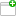This comment was attached:

arxcrash-11918-2020647432
#3
Comment posted byCrashBot
Jul 29, 10:20
A file was uploaded. cfg.iniThis comment was attached:

arxcrash-11918-2020647432
#4
Comment posted byCrashBot
Jul 29, 10:20
A file was uploaded. maps.txtThis comment was attached:

arxcrash-11918-2020647432
#5
Comment posted byCrashBot
Jul 29, 10:20
A file was uploaded. crash.txtThis comment was attached:

arxcrash-11918-2020647432